## Ti-Nspire: Differentials Step by Step

To solve Differentials such as this one:

We use Calculus Made Easy at www.Tinspireapps.com , select Differentials and enter as follows

Problem solved! Works for any Differential problem.

## TiNspire CX : Piecewise defined function

To solve problems involving piecewise defined functions with your TiNspire CX CAS use Calculus Made Easy at www.TiNspireApps.com and scroll down to option 9 :

Next, enter the 2 “pieces” of the function in the top box and the start/end point in the 2. box as follows :

Now scroll down to check if the piecewise defined is continuous or not. Here it is since the 2 one-sided limits match at x=2 .

## TiNspire CX Limit Solver

Use belows Limit Solver when asked to find a constant for which a limit exists. Ex: What is a in (x^2+x-a) /(x-2) as x approaches 2?

To find the constant a for which the limit exists using the TiNspire CX CAS use Calculus Made Easy at www.TinspireApps.com , select option 6 in the Limits menu :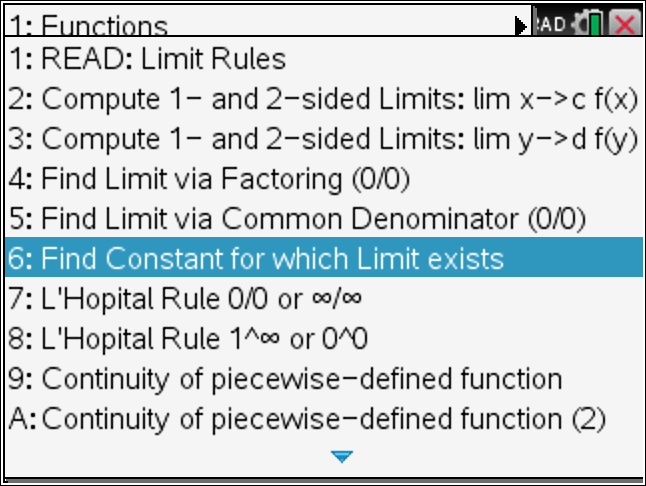When entering the above example, we find the Limit to be 5 when the constant a=6 .

## Partial Derivatives using the TiNspire Cx CAs

Finding Partial Derivatives using the TiNspire can easily be done using Calculus Made Easy at www.tinspireapps.com as follows: In the Menu select Multivariable Calculus, then select Partial Derivatives and Gradient:

Next enter the given function using x and y as variables:

The two partial derivatives are highlighted , the 2. partial derivatives are found shown below.

You do have the option to evaluate the partial derivatives by entering x0 and y0 values in the 2. box as shown below :

Posted on Categories calculus

## Tangent Plane – Step by Step – using the TiNspire CX

QUESTION:  Find an equation of the tangent plane to the surface
z=3x^4+9y^4+7xy at the point (3,3,1035).

SOLUTION: Start Calculus Made Easy , go to the Multivariable Calculus in the menu.

There, enter as shown below :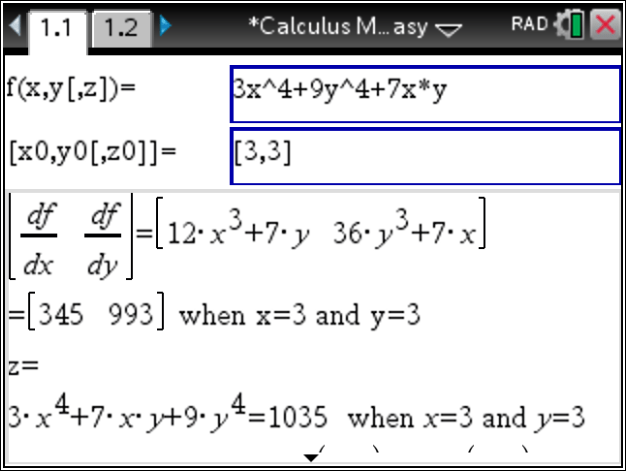The steps are shown in the box below: partial derivatives are computed and evaluated. And the function is evaluated at the given point . Thus, the Tangent Plane is derived. Additionally, a line normal to the plane and a normal vector are found.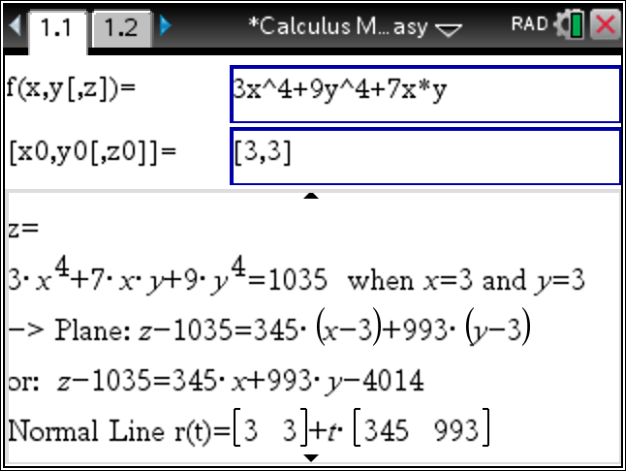Thus, finally
Posted on Categories calculus

## 2. Fundamental Theorem of Calculus using the TiNspire – Step by Step

Applying the Fundamental Theorem of Calculus using the TiNspire – Step by Step – can easily be done using Calculus Made Easy at www.TiNspireapps.com . Here is an example:

Just enter the given function f(t) which is the integrand.

Next, enter the upper bound of the given definite integral.

Then , Calculus Made Easy provides you with the Theorem and also applies it to show the correct answer.

Steps for the computation can be seen below: First the integrand is integrated and evaluated using the given bounds . Lastly, differentiation yields that final answer.

Posted on Categories calculus, tinspirecx

## TiNspire : Volume of Solids of Revolution using Disk, Washer and Shell Methods

Computing the Volume of a Solid of Revolution using the TiNspire CX CAS can easily be done – step by step – using the Calculus Made Easy at www.TinspireApps.com .

Here is how.

Then this pops up :

Now, enter the given function in the top box and the given interval below. Automatically, the answer will show in the bottom with correct integral setup and the correct answer.

Similarly, when using the Washer Method for two functions we have:

Finally, the Shell method works the same way :

In conclusion, the just like all other Calculus topics finding the Volume of Solids of Revolution using the Disk , Washer and Shell Methods can be done easily using Calculus Made Easy at www.TiNspireApps.com .

Posted on Categories calculus, tinspirecx

## TiNspire Users in Finnland comments

Kuulin koulussa 3 ihmisen ryhmän puhuvan Calculus kurssista miten hän läpäisi kurssinsa sovelluksen, avulla joka näytti kaikki välivaihe ratkaisut.
Luokkakaveri oli puhunut myöskin ohjelmasta, jonka nimi oli Calculus made easy, mikä näytti kuulemma välivaihe ratkaisut.

Yhden matikan kurssin reputtaneena ja toisenkin ehkä myöskin, turhautumiseni matematiikka kolmannen matikan Calculus kurssiin alkoi olemaan stressaavaa.
Päätin ostaa Calculus Made easy ohjelma TI-Nspire CX CAS laskimeen, tämä ohjelman avulla olen onnistunut tekemään kotitehtäviä jotka, ovat olleet haastavia minulle!
Erittäin hyödyllinen ohjelma niille jotka eivät ole keskiverto tasoa matikan laskemisessa.

Posted on Categories calculus, tinspirecx

## Ti-Nspire : Step by Step Definite Integrals

Computing definite Integrals using the 1. Fundamental Theorem of Calculus can be achieved using Calculus Made Easy at https://www.tinspireapps.com/?a=CME

Use option 8 in the INTEGRATION menu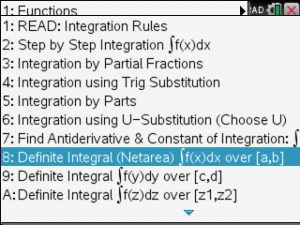Enter function f(x) and bounds [a,b] as shown below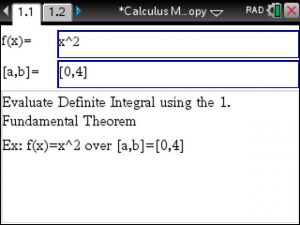View the Integration steps, here, the Power Rule is applied.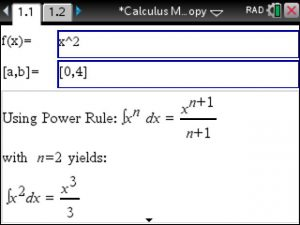Lastly, plug the bounds into the above Antiderivative, subtract and that’s it!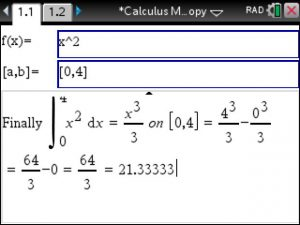Posted on Categories calculus

## Tinspire CX CAS : Potential Function and Conservative Vector Field – Step by Step

To Find the Potential Function for a given Vector Field such as the one at http://tutorial.math.lamar.edu/Solutions/CalcIII/ConservativeVectorField/Prob4.aspx

use the Vector Calculus Made Easy app at https://www.tinspireapps.com/?a=VCME  , go to option 3,4

and enter the Vector Field as: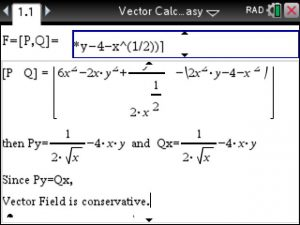Since the 2 partial derivatives match the Vector Field is conservative

and the Potential Function is found as shown below:Posted on Categories calculus, vector